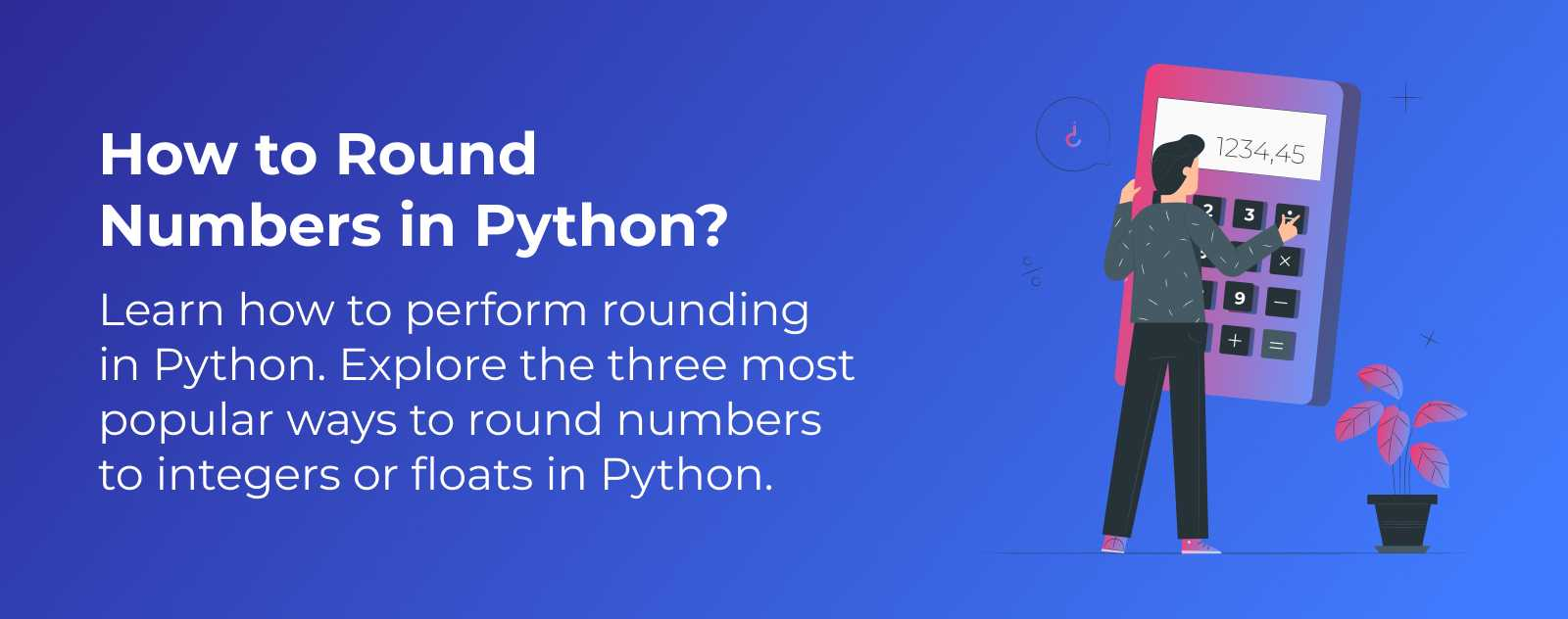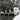# How to Round Numbers in Python?

Article updated on Monday, November 27, 2023.In Python, rounding is done using the `round()` function.

The `round()` function rounds a decimal number to the nearest integer. It also rounds floating-point numbers to a specified decimal place.

Let’s see a practical example directly:

``````number = round(42.21)
print(number)``````

This will assign the value to the `number` variable:

``42``

To round up or down, you can use the `ceil()` and `floor()` functions from Python’s `math` module.

``````import math

up = math.ceil(42.1)
down = math.floor(42.1)

print(up)
print(down)``````

This will assign the values to the `up` and `down` variables:

``````43  # up
42  # down``````

## How to Round to a Decimal Place in Python?

The `round()` function takes a second parameter: the number of decimal places to round to.

``````number = round(42.214284, 2)
print(number)``````

This will assign the value to the `number` variable:

``42.21``

## How to Round Up in Python?

Python’s `math` module’s `ceil()` function rounds a number to its next highest integer.

``````import math

number = math.ceil(42.000001)
print(number)``````

This will assign the value to the `number` variable:

``43``

The `ceil()` function rounded our number up to the next integer even though `42.000001` is closer to 42 than to 43.

## How to Round Down in Python?

Python’s `math` module’s `floor()` function rounds a number to its next lowest integer.

``````import math

number = math.floor(42.999999)
print(number)``````

This will assign the value to the `number` variable:

``42``

The `floor()` function rounded our number down to the next lowest integer even though `42.999999` is closer to 43 than to 42.

## How Will You Perform Rounding?

Congratulations! You now know the three native ways in Python to round numbers 🎉

We’ve covered rounding a number to the nearest integer, rounding up or down, and rounding to a specified number of decimal places.

Feel free to sign up for the email list below if you want more programming tips.Hey, I'm Thomas 👋 Here, you'll find articles about tech, and what I think about the world. I've been coding for 20+ years, built many projects including Startups. Check my About/Start here page to know more :).